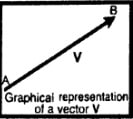How can vectors quantities can be represented graphically?

Difficulty: Medium

Symbolic Representation of a vector:

To differentiate a vector from a scalar quantity, we generally use bold letters to represents vectors quantities such as F, a, d or a bar or an arrow over the symbols such as $\bar{F},\bar{a},\bar{d} \: or \: \overrightarrow{F},\overrightarrow{a} ,\overrightarrow{d}$

Graphical Representation of a vector:

A straight line is drawn with an arrowhead at one end. According to some suitable scale, the length of a line represents the magnitude and the arrowhead gives the direction of the vector.